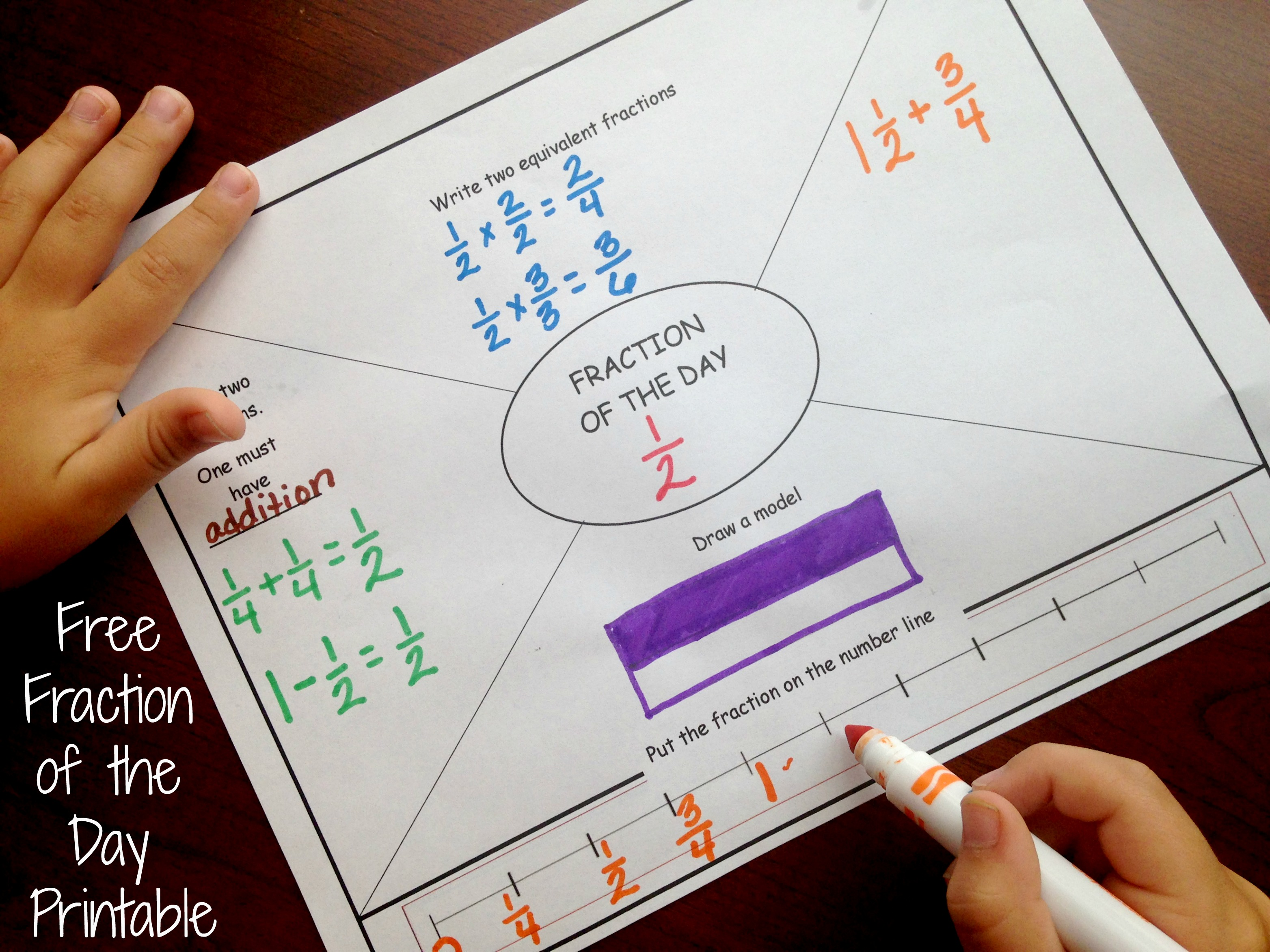Home » Math » How to Teach Fractions Using Number Lines

How to Teach Fractions Using Number Lines

Teaching fractions on a number line? Grab this free printable that challenges students draw number lines to help them Develop Fraction Sense Using Number Lines.

It’s week three of our Developing Fraction Sense series.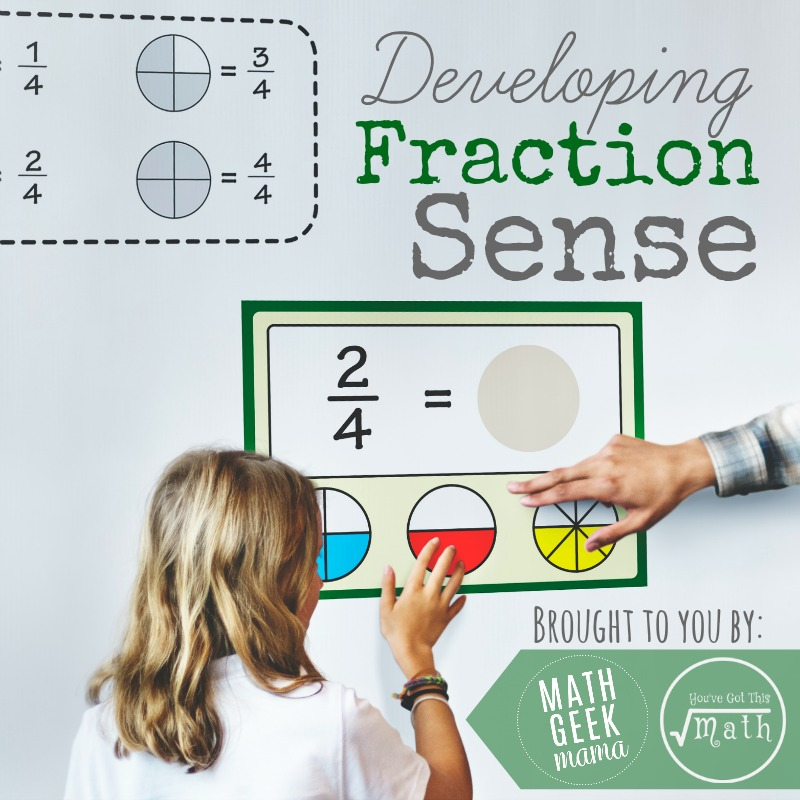We began with a look at Numerators and Denominators….

Get it here

Then, Math Geek Mama talked about using benchmarks….

Get That Post Here

Today we are going to discuss three ways for teaching fractions on a number line.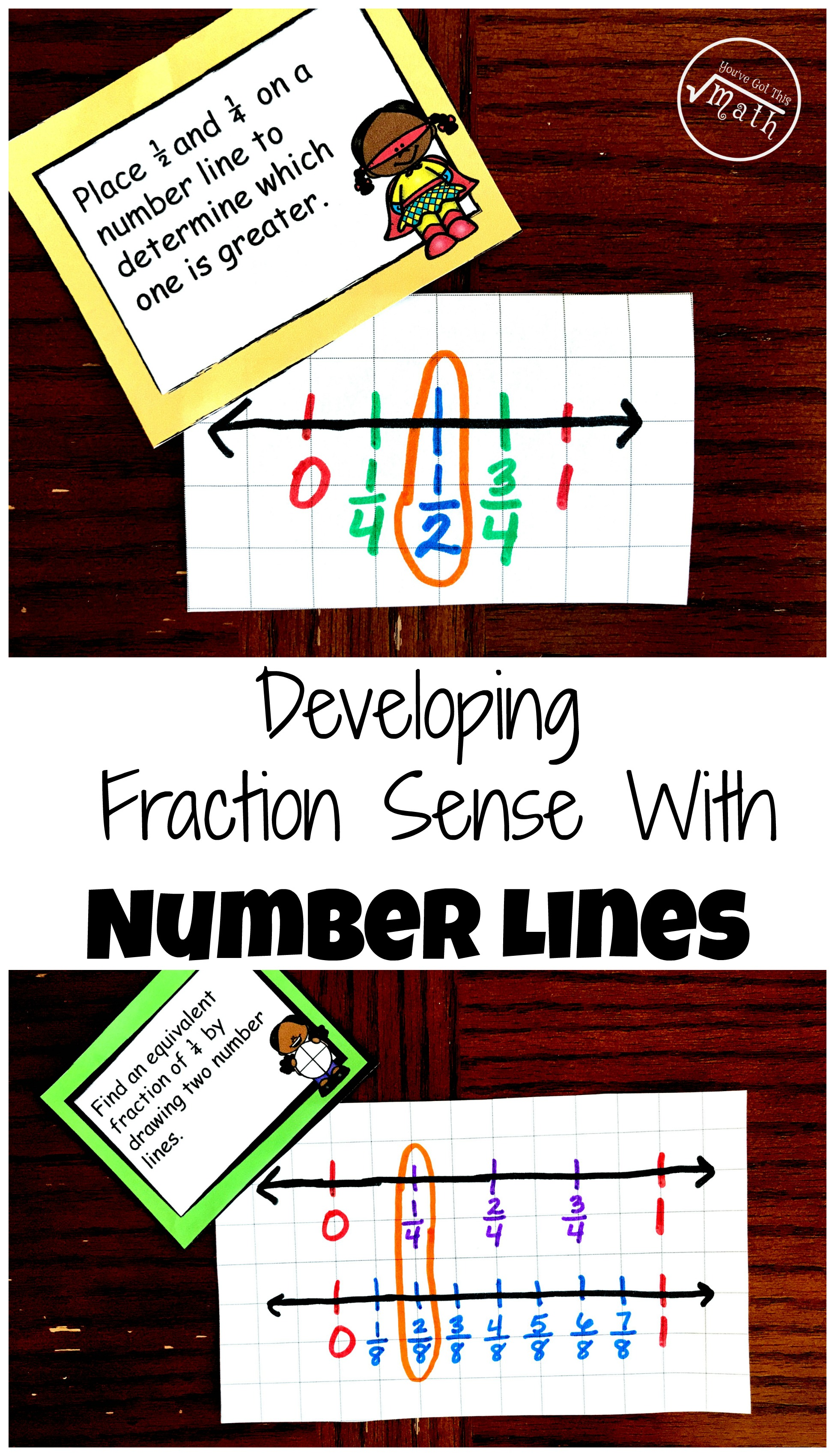This post may contain Amazon links and links to other bloggers. There is no extra cost to you, but if you choose to purchase, I receive a small percentage. It helps with the upkeep of You’ve Got This Math and provides for my family.

Number lines are a huge part of building number sense in lower grades, and it can be just as effective with fractions.

Here are three ways to use them….and this is just the start. There are so many other ways!

Understanding Numerators and Denominators

For children to draw a number line and correctly label it with fractions, they must know what the numerator and denominator represent.

If you are asking a child to place 3/5 on a number line, they have to understand their number line must be divided into five equal parts between the whole number 0 and 1. Next, they have to recognize that the third line from the 0, is where 3/5 is located.

Children also get the extra benefit of seeing that a fraction is between the numbers 0 and 1. It drives in the fact in a new way that a fraction does not equal one whole. You can also do this activity with mixed numbers to help children see that a mixed number also falls between two whole numbers.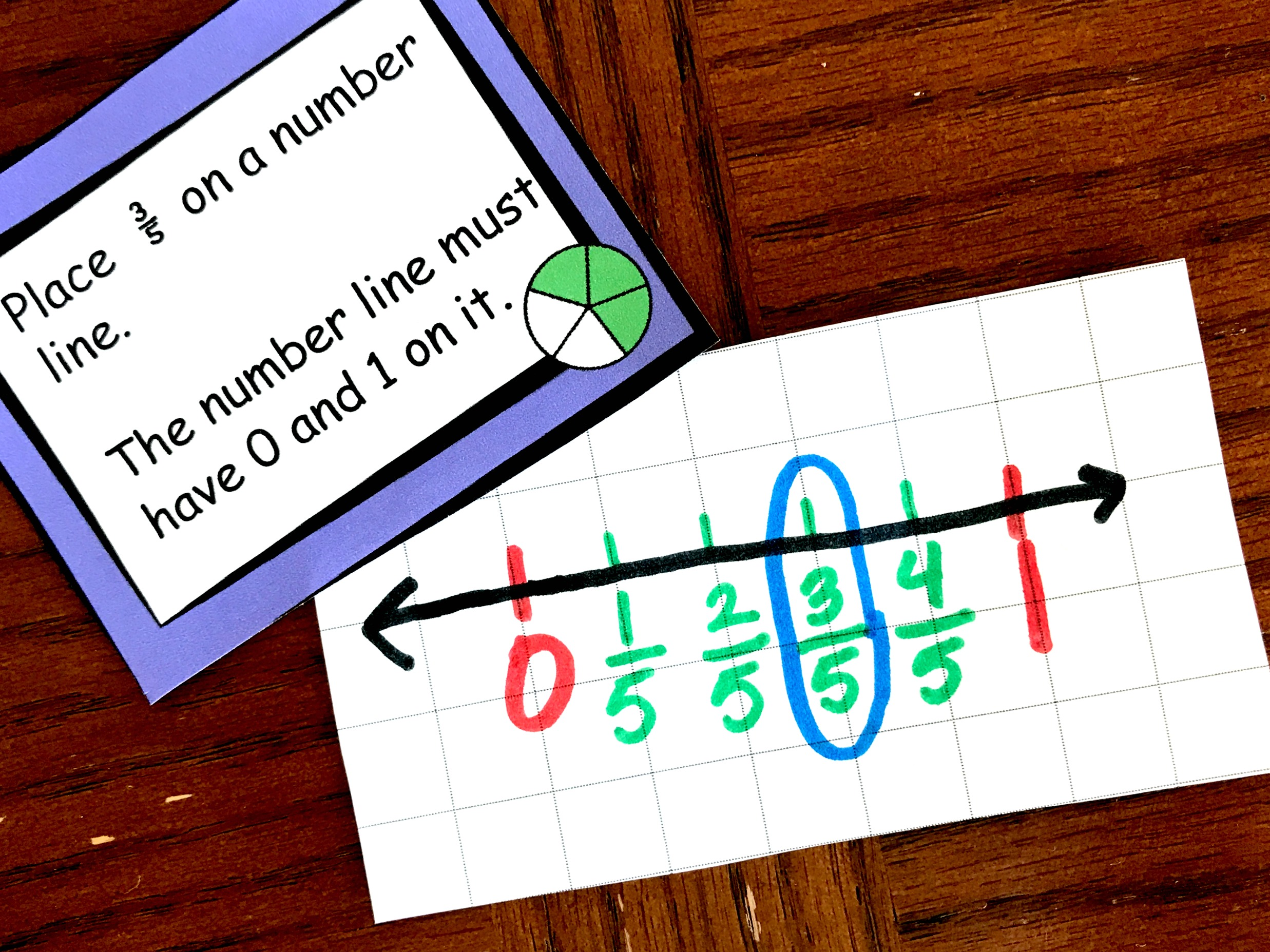Equivalent Fractions

Another way to use number lines is to teach equivalent fractions.

If you plan on teaching equivalent fractions with this method, you may need to provide direct instruction first…..and some grid paper.

1. To begin, inform your students that both number lines will have a 0 and a 1 in them and that each number line must have the same amount of spaces. The zero and the one from the second line must be placed directly underneath the zero and one from the first number line.
2. Next, ask them what part of the fraction will help them determine how many grid spaces each set of number lines will have. (The denominator)
3. Now comes the fun part! Figuring out how many grid spaces each line will have. Begin by having the students “skip count” by the denominator and choose one of the multiples. (This is an introduction for Least Common Mulitple). Now the children draw their number lines with that many grid spaces in each line.
• For example, if you are finding an equivalent fraction for 1/2 they could create two lines that have 4,6,8,10, etc spaces.
4.  Following that, the children write the fractions on the number line.
• Let’s say the children choose the multiple 4. For the first line, the child would have to realize that they have to take the number of spaces and divide it by the denominator. Four divided by two equals two, so they would go over 2 spaces from the zero, place and place a line for the fraction 1/2.
5. Finally, any fraction that is directly underneath a fraction on the first number line is an equivalent fraction.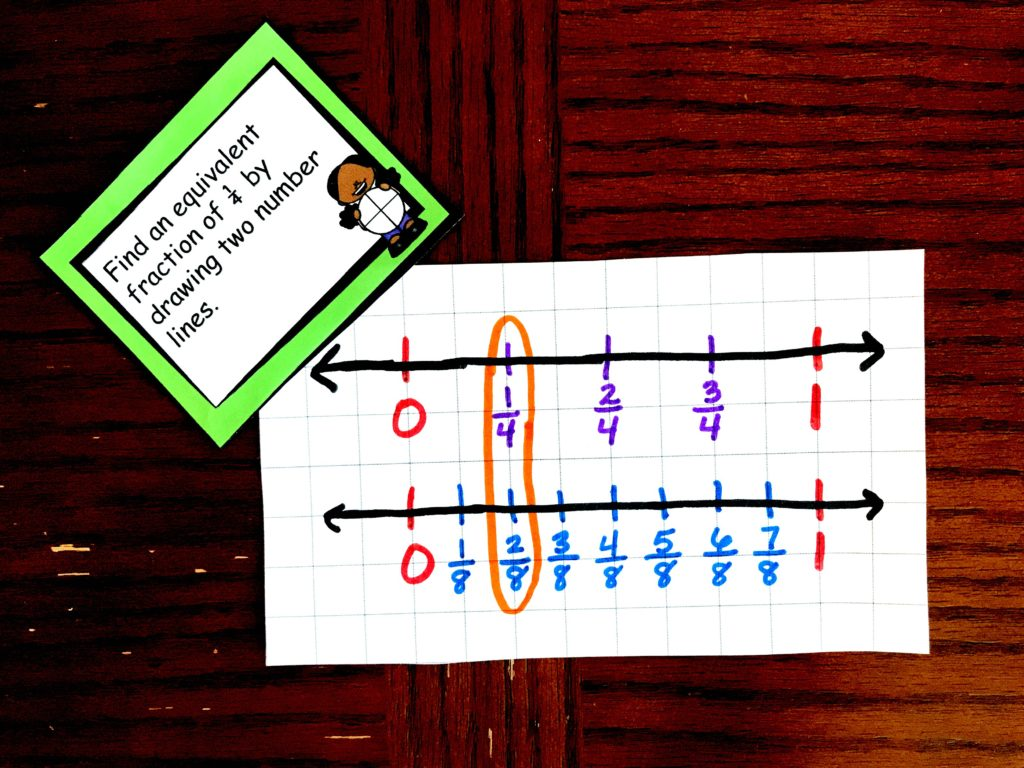Using Number Lines to Teach Comparing Fractions

Comparing fractions on a number line is very similar to finding equivalent fractions on a number line. The children begin by finding the least common multiple of the two fractions and creating two lines with that many spaces. Once the place the fractions on the number line it is easy to see which fraction is greater.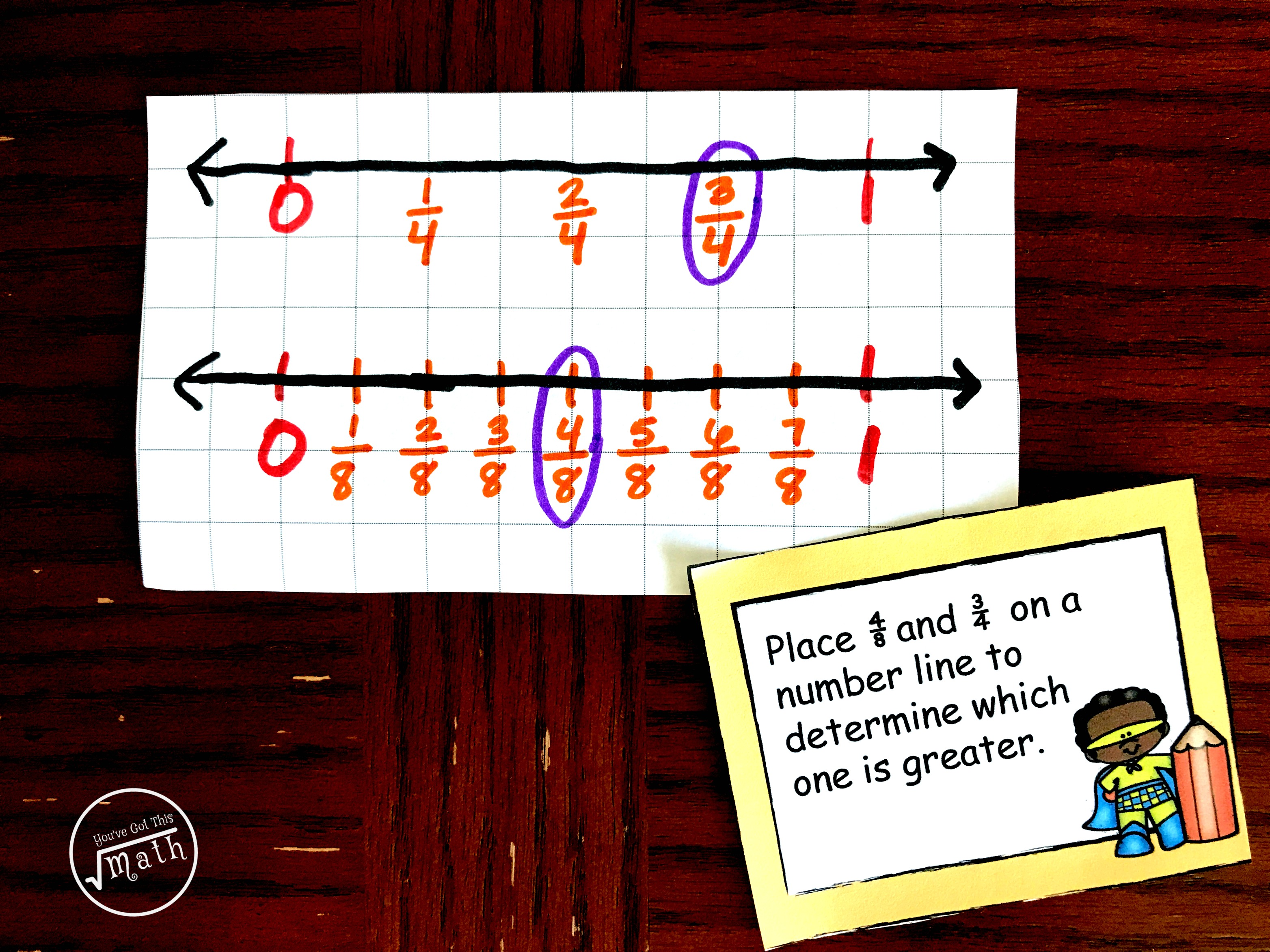As an extra challenge, a child can place the fractions on one number line. When doing this it may be easiest to place the fraction with the smallest denominator on it first, and then go back and put the fraction with the largest denominator on the number line.Free Printable To Develop Fraction Sense Using Number Lines

I’m a firm believer in having children create their own number lines. It requires a massive amount of thinking and strategizing, which develops fraction sense, and this printable provides simple challenges that will get children drawing and thinking.

This printable has three different challenges….

1. Challenges Focusing on Placing Fractions on a Number Line
2. Equivalent Fraction Challenges
3. Comparing Fraction Challenges

What you Need For Teaching Fractions On A Number Line

To prepare for children drawing number lines, you need to have tons of grid paper available. You can print, purchase a pack, or my favorite…..have math journals with grid paper in it. (My colleagues and I loved being able to provide these to our kiddos, and at least three times a week our kiddos were solving problems and modeling them in their journals).

Next grab some pencils, colored pencils (if desired) and the printable and you are ready to go.

Drawing number lines can be frustrating for some children but keep at it. The learning that comes from it is worth every struggle. You will be glad you taught it this way, and your kiddos will grow as mathematicians too!!

You’ve Got This

Rachel

You May Also Like